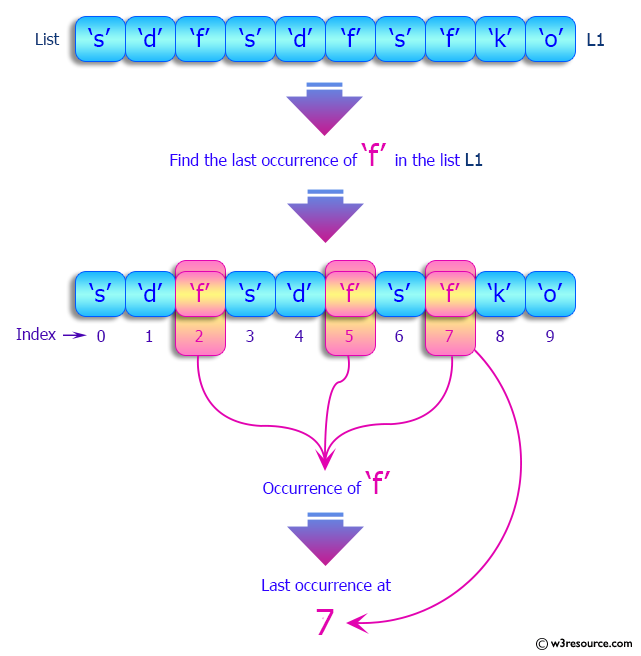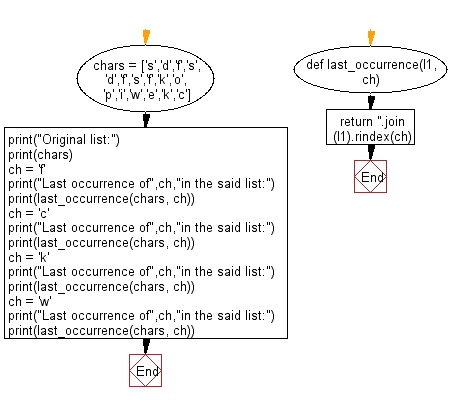﻿ Python: Find the last occurrence of a specified item in a given list - w3resource# Python: Find the last occurrence of a specified item in a given list

## Python List: Exercise - 162 with Solution

Write a Python program to find the last occurrence of a specified item in a given list.

Sample Solution:

Python Code:

``````def last_occurrence(l1, ch):
return ''.join(l1).rindex(ch)

chars = ['s','d','f','s','d','f','s','f','k','o','p','i','w','e','k','c']
print("Original list:")
print(chars)
ch = 'f'
print("Last occurrence of",ch,"in the said list:")
print(last_occurrence(chars, ch))
ch = 'c'
print("Last occurrence of",ch,"in the said list:")
print(last_occurrence(chars, ch))
ch = 'k'
print("Last occurrence of",ch,"in the said list:")
print(last_occurrence(chars, ch))
ch = 'w'
print("Last occurrence of",ch,"in the said list:")
print(last_occurrence(chars, ch))
```
```

Sample Output:

```Original list:
['s', 'd', 'f', 's', 'd', 'f', 's', 'f', 'k', 'o', 'p', 'i', 'w', 'e', 'k', 'c']
Last occurrence of f in the said list:
7
Last occurrence of c in the said list:
15
Last occurrence of k in the said list:
14
Last occurrence of w in the said list:
12
```

Pictorial Presentation:Flowchart:## Visualize Python code execution:

The following tool visualize what the computer is doing step-by-step as it executes the said program:

Python Code Editor:

Have another way to solve this solution? Contribute your code (and comments) through Disqus.

What is the difficulty level of this exercise?

Test your Python skills with w3resource's quiz

﻿

## Python: Tips of the Day

Floor Division:

When we speak of division we normally mean (/) float division operator, this will give a precise result in float format with decimals.

For a rounded integer result there is (//) floor division operator in Python. Floor division will only give integer results that are round numbers.

```print(1000 // 300)
print(1000 / 300)```

Output:

```3
3.3333333333333335```